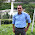VHDL coding tips and tricks: Clock Frequency converter in VHDL

## Wednesday, March 9, 2011

### Clock Frequency converter in VHDL

In FPGA designs, there are situations where you want a clock signal with a small frequency(or high time period). But in most of FPGA boards the frequency of the crystal oscillators available are of the range of tens of MHz.

One solution to the above problem is to take the high frequency clock available on board and convert it to a lower frequency clock. This is called frequency down conversion. I have shared a code here for a general purpose clock down converter.

The entity clk_gen takes a high frequency clock, Clk and an integer value divide_value as inputs and produces the converted clock at Clk_mod. The divide_value is defined as follows:

divide_value = (Frequency of Clk) / (Frequency of Clk_mod).

For example if you want to convert a 100 MHz signal into a 2 MHz signal then set the divide_value port as "50".

Without much further explanation I will give you the code:

library IEEE;
use IEEE.STD_LOGIC_1164.ALL;
use IEEE.NUMERIC_STD.ALL;

entity clk_gen is
port(   Clk : in std_logic;
Clk_mod : out std_logic;
divide_value : in integer
);
end clk_gen;

architecture Behavioral of clk_gen is

signal counter,divide : integer := 0;

begin

divide <= divide_value;

process(Clk)
begin
if( rising_edge(Clk) ) then
if(counter < divide/2-1) then
counter <= counter + 1;
Clk_mod <= '0';
elsif(counter < divide-1) then
counter <= counter + 1;
Clk_mod <= '1';
else
Clk_mod <= '0';
counter <= 0;
end if;
end if;
end process;

end Behavioral;

The testbench code used for testing the code is given below:

LIBRARY ieee;
USE ieee.std_logic_1164.ALL;
USE ieee.numeric_std.ALL;

ENTITY testbench IS
END testbench;

ARCHITECTURE behavior OF testbench IS

signal clk,clk_mod :  std_logic;
signal divide_value :  integer;
constant clk_period : time := 10 ns;

begin
-- Component Instantiation
uut: entity work.clk_gen PORT MAP(
clk => clk,
clk_mod => clk_mod,
divide_value => divide_value );

simulate : process
begin
divide_value <= 10;  --divide the input clock by 10 to get 10(100/10) MHz signal.
wait for 500 ns;
divide_value <= 19;  --divide the input clock by 19 to get 5.3(100/19) MHz signal.
wait;
end process;

clk_process :process  --generates a 100 MHz clock.
begin
clk <= '0';
wait for clk_period/2;  --for 5 ns signal is '0'.
clk <= '1';
wait for clk_period/2;  --for next 5 ns signal is '1'.
end process;

END;

Note :- The code was simulated and synthesised successfully using Xilinx Webpack version 13.1. It should work fine under other tools as well.

#### 6 comments:

1.Again, as in previous posts, your code results in clocks being taken of the low-skew global clock paths in the device. This is bad and as the previous commenter pointed out, WILL give problems at high frequencies.

A better solution is to use a downsampled clock enable on your blocks. In this way the clock is still on the low-skew paths and you will make timing at high frequencies.

2.Very good post. Thanks again. For odd integer clock division the duty cycle isn't 50% though.

3.how can we write a code for the conversion of 1mhz to 1khz.

1.divide_value = (Frequency of Clk) / (Frequency of Clk_mod).

so divide_value = 1000000/1000 = 1000.

4.Hi, thanks for your examples.
I simplified your code and added reset signal as below:

architecture Behavioral of main is
signal clk_div : STD_LOGIC := '0';
signal scnt : INTEGER := 2;
begin
clk_div_proc: process(clk, rst)
variable count : INTEGER := 0;
begin
if rst = '1' then
clk_div <= '0';
count := 0;
elsif( rising_edge(clk) ) then
if(count < scnt/2-1) then
count := count + 1;
else
clk_div <= not clk_div;
count := 0;
end if;
end if;
end process;

end Behavioral;

Best regards.

5.Hi i have 48MHz clock frequency anda i have to conver it to 20 MHz.
But 48/20= 2.4 so it is not an integer what can be done to do this?

Thanks for your help